Processing Color Images

6.1 The Light Spectrum and Human Perception

Light as Electromagnetic Energy. Light can be considered as waveforms or rays of particles (photons) because of the extremely small wavelength (as the wavelength l becomes smaller, the wave behavior becomes more like that of a particle). This means that the frequency f is extremely high because

f = c/l, l = c/f, c = lf                          (6.1)

where c is the speed of light. In vacuous space, c = 3x108 m/sec. Thus f becomes (m/sec)/m = 1/sec. (the number of wavelengths per second).

A waveform can be represented as a function r(x,y,,t) as it moves across an area in x and y through time t at a wavelength of . We call r the radiant flux per area wavelength, or irradiance per wavelength.

Perception of Light by the Human Eye. The wavelengths of light energy affect the receptors in the back of the human eye. There is not enough strength of this effect to pass the threshold for perception except for wavelengths of a limited range. However, from about 700 nm (red) to 400 nm (violet), there is perception, witht the most efficient detection being in the middle at about 546 nm (green). The cones (color sensors) in the center region of the back of the eye require higher energy for detection, while the rods (grayscale sensors) in the region outside of the cones are extremely sensitive to light (they give us peripheral vision and vision in faint light). Figure 6.1 presents the electromagnetic spectrum and the visible light portion. Figure 6.2 shows that the human eye is most sensitive green. It is sensitive also to small changes in grayscale and is less sensitive to small changes in color, which affects the way we need to process color images.

Figure 6.1. The Electromagnetic Spectrum and Visible Spectrum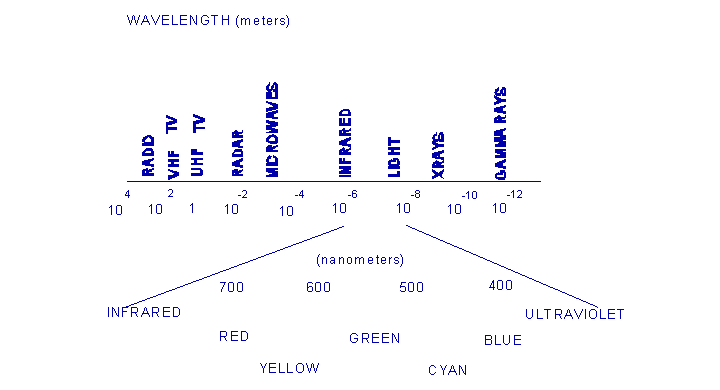Figure 6.2. Human perception of light energy. Figure 6.3. Additive and subtractive colors.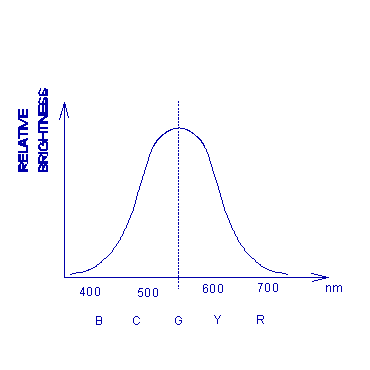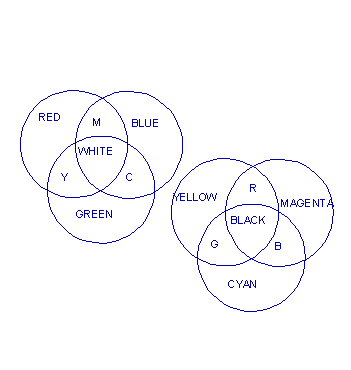6.2 The RGB and CMY Color Models

The RGB Additive Color Model. The three primary (independent) colors red, green and blue (RGB) can be added in various combinations to compose any color in the spectrum. Figure 6.3 demonstrates the additive property. R is lower in the visible frequency spectrum (longer wavelength), G is in the middle and B is higher. Below R is infrared, while above B is ultraviolet. Neither infrared nor ultraviolet can be detected by the human eye. The wavelengths in nanometers (a nm is 10-9 meter) are

Red: = 700 nm Green: = 546 nm Blue: = 435.8 nm

G + B = Cyan (C), B + R = Magenta (M), R + G = Yellow (Y), R + G + B = White (W)

In the RGB model, CMY are secondary colors. Figure 6.4 shows the RBG color cube. Each of R, G and B is standardized to take values between 0 and 1. The origin (0,0,0) is Black because there is no energy (0 intensity), while the point (1,1,1) is pure white because it has equal parts of R, G and B at maximum value.

We can normalize the colors via

r = R/(R+G+B), g = G/(R+G+B), b = B/(R+G+B)          (6.2)

r + g + b = 1                                        (6.3)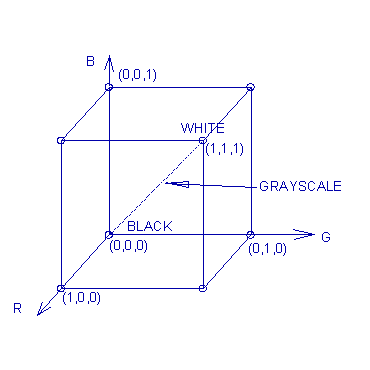Figure 6.4. The RGB color cube.

The CYM Subtractive Color Model. We can also take CYM (Cyan, Yellow and Magenta) as the primary colors, in which case RGB become the secondary colors. Molecules in a material of a subtractive color absorbs energy at the frequency of the subtractive colors and thus subtracts that color. Figure 6.3 also shows the subtractive model. Paints of colors C, Y and M can be mixed to form

C + M = Blue, C + Y = Green

M + Y = Red, C + M + Y = Black

Conversion Between RGB and CMY. Given the RGB or CMY coordinates of a pixel color, it can be respectively converted into CMY or RGB via

C = 1 - R

M = 1 - G                                               (6.4)

Y = 1 - B

R = 1 - C

G = 1 - M                                              (6.5)

B = 1 - Y

CMY is useful in special situations. For example, many color printers use the subtractive model and have built-in logic for converting RGB to CMY.

6.3 HSI and RGB-HSI Transformations

The HSI Model. The hue, saturation and intensity model of color takes advantage of the way humans perceive color. Hue (H) is the color perceived due to the wavelength. Saturation (S) is the degree to which the color is pure, or free, from white light (grayscale) dilution, or pollution. High saturation means that the color is highly pure, while low saturation means that there is a lot of dilution with white light that is made by the presence of R, G and B primary components. H and S taken together provide the chromaticity, or color content of a pixel. Intensity is the brightness, or energy level of the light and is devoid of any color content. Together, HSI (hue, saturation and intensity) make any color and intensity of light. Figure 6.5 displays the chromaticity triangle. Any point P on the triangle represents a color.

The boundary of the chromaticity triangle represents pure color with no pollution by white light. Thus there is only a single wavelength of light present along the boundary (a color that can be made by two primary colors). Points closer to the middle have proportionately more pollution by white light (the minimum amount of a third primary color increases) so that at the middle point, there is nothing but white light (equal amounts of R, G and B). The proportion of the distance from the center point to P of the distance from the center point to the boundary through P is the saturation.

Figure 6.5. The chromaticity triangle. Figure 6.6. The HSI color solid.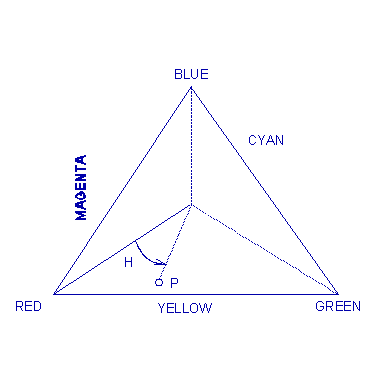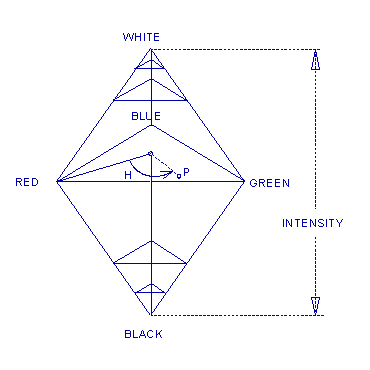The hue of a color at point P is the angle of P from the line that connects the center point to the point at pure Red. Starting at Red and traversing the boundary in the counterclockwise direction, we go to Yellow (60), then Green (120), Cyan (180), Blue (240), Magenta (300) and then back to Red (360 or 0).

Figure 6.7. A slice of the color cube.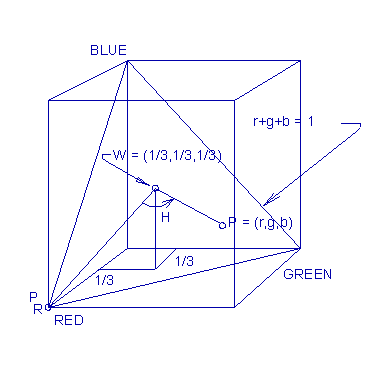The chromaticity triangle does not model the intensity, which is a fixed amount for each triangle. We can see from Figure 6.6 that the HSI color solid provides the intensity as well as the chromaticity. For any intensity level, a cross-section is a chromaticity triangle.

Conversion between HSI and RGB. The reason we desire to convert the colors of the image pixels from RGB to HSI is so that we can process the intensity part I to obtain the new intensity I~, then convert HSI~ back to RGB for display. That way we do not change the colors but can sharpen, smooth, or otherwise process the image.

RGB Intensity. Each of R, G and B is standardized to take values between 0 and 1, with a maximum total intensity of 1. Thus we put

I = (R + G + B)/3, (0 I 1)                            (6.6)

RGB Saturation. White light is caused by equal amounts of each of R, G and B. If we take the minimum one of these and subtract that amount from each one, what is left has only two color components instead of three and thus has no white light pollution. Therefore, it is fully saturated and represents a single wavelength (see Figure 6.2) or a combination of only two primary colors. The proportion of light that remains after such subtraction is the saturation. Thus

S = 1 - min{R,G,B}/I = [I - min{R,G,B}]/I               (6.7)

RGB Hue. The HSI chromaticity triangle, for a given intensity, corresponds to a plane through the RGB color cube. Figure 6.7 shows the triangle of points (r,g,b) = (R/(R+G+B), G/(R+G+B), B/(R+G+B)) that satisfy r + g + b = 1. The center point W has coordinates (1/3,1/3,1/3). We can find the angle H = for any point P on the triangle by the geometry of the triangle. Hue is a value between 0 and 360.

We consider first the case along the lower half of the HSI chromaticity triangle where B G (a line from R to Cyan). Let P be the point as shown in Figure 6.5 and W be the center. Upon projecting the vector P - W onto the vector (line) PR - W via the cosine formula for the dot product, we have

(P - W)@(R - W) = |P - W||PR - W|cos(H)                 (6.8)

where H is the angle.

From

|P - W| = sqrt{sq(r - 1/3) + sq(g - 1/3) + sq(b - 1/3)} (6.9)

and r = R/(R+G+B), g = G/(R+G+B), b = B/(R+G+B), we obtain

|P - W| =

sqrt{9(sq(R)+sq(G)+sq(B)) - 3sq(R+G+B)/[9sq(R+G+B)]     (6.10a)

|P - R| = sqrt(2/3)                                     (6.10b)

so that

(P - W)@(R - W) = {2R - G - B}/{3(R+G+B)}              (6.11)

From Equation (6.8) we solve for

cos(H) = (P - W)@(R - W) / |P - W||R - W|               (6.12)

Thus

H = arccos{(P - W)@(R - W) / [|P - W||R - W|]

= arccos{(1/2)[(R-G) + (R-B)] /sqrt[sq(R-B) + (R-B)(G-B)]}

B <= G                                                  (6.13a)

When the color is in the upper half of the chromaticity triangle, we must
subtract this angle from 360 to obtain the hue.

H = 360 - H, B > G                                      (6.13b)

Conversion from HSI to RGB. To convert in the opposite direction, we consider
the cases where the point P is in one of the following regions of the
chromaticity triangle: H <= 120, 120 <= H <= 240, or 240 <= H <= 360. It can
be verified that the following do the inverse conversion.

H <= 120:       b = (1/3)(I - S)
r = (1/3){I + Scos(H)/cos(60-H)}
g = 1 - (r + b)                         (6.14a)

120 <= H <= 240:      r = (1/3)(I - S)
g = (1/3){I + Scos(H)/cos(60-H)}

b = 1 - (r + g)                         (6.14b)

240 <= H <= 360:      g = (1/3)(I - S)

b = (1/3){I + Scos(H)/cos(60-H)} r = 1 - (g + b) (6.14c)

6.4 Processing Color Images

Smoothing Hue and Saturation. Because the human has higher sensitivity to the intensity component and relatively less sensitivity to color, we convert to HSI and perform different types of processing on I and the color components. The I component image fI(m,n) can be processed as any other grayscale image, and so we can sharpen it, equalize its histogram, stretch the contrast, remove noise from it via standard grayscale methods, or process it by other grayscale methods.

The color is not disturbed by the intensity processing. A common type of processing for the color components is to pass the hue and saturation through lowpass filters to remove the color noise. This is done effectively with a median filter. Slight blurring of hue and saturation is not noticeable when the processed image is displayed.

Generally, we have the 3 images fR(m,n), fG(m,n) and fB(m,n) of the R, G and B values, respectively. These are converted into fH(m,n), fS(m,n) and fI(m,n) (or fY(m,n), fI(m,n) and fQ(m,n): see the section below). Then the appropriate component images are processed and converted back to the RGB component images for display.

Color Balance. Color may be out of balance due to digitizing during capture or scanning, or due to the photoprocessing of photographic film. The imbalance is caused by shifting the color from what it should be. The most important test is to examine objects that should be gray to see if they have color, and if so, then the color is out of balance. A second test is to examine objects that have highly saturated colors to see if the colors are incorrect.

The usual situation is that the balance can be restored by linear grayscale transformations on two of the R, G, and B colors values, as only two of these need to be changed to match the third. By taking the average values for each of R, G and B over an object of dark gray, the average values µR, µG, and µB should be equal. The same holds true for the average values of the R, G and B values over a region of light gray. Linear transformations can be designed for two of the colors to change the average values so that they are all equal both on a dark gray object and on a light gray object. The transformation for adjusting the means is given in Section 2.7 of Chapter 2.

Color Processing. The most common type of color processing of images is the grayscale processing of the intensity values when the image is converted from RGB to HSI, and then converting back to RGB with the new processed I~ values. This type of processing can also be done with the YIQ model, described below, where Y is the intensity (I and Q provide the color). However, the colors may be changed to enhance the image, and indeed, they sometimes strongly need to be changed. Some of this can be done using RGB, while other types of processing require HSI or YIQ.

The colors can be made stronger (purer) by increasing the saturation, S, by multiplying it by a number greater than 1. Multiplying by a number less than 1 causes the color to become weaker. Only those pixels with significant saturation should be changed because the increased saturation of colors that are too close to the point W can cause color imbalance.

Colors that contain larger proportions of R than of G and B are called warm, while those that contain more B than R or G are called cool. Hues can be shifted by adding or subtracting H to the H values to make the colors "warmer" or "cooler." The increment should be small so as to not yield an undesirable appearance.

6.5 The YIQ Color Model

The YIQ Model for Commercial TV Broadcasting. The Electronic Industries Association (EIA) defined the RS-170 specifications for monochrome television, using techniques that were defined in the 1930s. The image was painted on a cathode ray tube (CRT) with a raster scan of lines to compose a picture of 525 lines per frame, interlaced so that the even numbered lines were written 30 times per second and similarly for the odd numbered lines, so that there was the illusion of 60 frames per second (60 half-frames, or fields) to avoid flicker. The aspect ratio was 4-to-3, with 646 square pixels per line. A few of the lines (11) were used for synchronization and were not actual lines of pixels. The signal was analog with a horizontal synchronizing pulse at the end of each line, and a vertical synchronizing pulse at the end of 525 lines, which indicates a return to the beginning of the frame. The 30 fields were written in 33.3 milliseconds (that is, a field every 1/30th of a second). The RS-170 signal ranges from -0.286 to 0.714 volts for a total difference of 1 volt, but the actual voltage that represents the brightness (intensity) from black to white varies from 0.143 to 0.714. Synchronizing pulses go down to -0.286 volts.

When commercial television broadcasting systems changed to color, there were still millions of monochrome TV sets in use. The color TV signal that was adopted retained the monochrome signal Y that would work with these monochrome receiver sets, but contained components I and Q for the color that could be used by the color TV receivers. Thus the YIQ model was initiated. The color TV system in the United States is known as the NTSC for the National Television Systems Committee that was accepted in 1953 and was adopted as the EIA RS-170A specification.

The brightness is the Y signal from the RS-170, but a color subcarrier waveform carries the chrominance. The hue is modulated in the waveform as a phase, while the saturation is carried as an amplitude. A color reference signal (the color burst) is added at the beginning of each video line. The composite signal is an analog waveform in a 4.2 MHz bandwidth centered on the channel frequency. The color subcarrier is at 3.58 MHz and is quadrature modulated onto the carrier wave. The bandwidth of the I component is 1.3 MHz and the Q bandwith is 0.6 MHz.

Y contains the luminance, or intensity, while I and Q contain the color information that is decoupled from luminance. Thus the Y component can be processed by grayscale methods without affecting the color content. For example, Y can be processed with histogram equalization. The situation is the same as processing with HSI.

Recall that the human eye contains sensors in the retina (inside surface of the back of the eyeball) that are of two types: i) color cones that receive the light ray more directly and require stronger signals (daytime vision); ii) and grayscale rods that are very sensitive and are used mostly in peripheral vision (and nighttime vision). Because the eye is more sensitive to changes in luminance than in colors, the Y signal contains a wider bandwidth (of Hz) than the I and Q for greater resolution in Y. Thus we can smooth I and Q to remove color noise and process the Y image fY(m.n) as we would any grayscale image.

Gamma Correction. The brightness (intensity) of the pixels is modeled as a constant times a power of the voltage plus a constant that is the blankout (black) level at which the screen goes black. The model is

brightness = cvg + b (6.15)

where c is a constant gain, v is the voltage of the signal and b is a level at which the color is still black in the CRT. Gamma is the exponential g.

The of a CCD (charged-coupled device) is 1.0, while that of a videocon type picture tube is 1.7. CRT tubes, including TV sets and video monitors, vary from 2.2 to 2.5. To avoid the necessity of having gamma correcting inside each TV set, the NTSC includes the gamma correction to be made before the signal is broadcast. Thus a gamma correction of 1/2.2 = 0.45 is made in the signal and then it is broadcast.

Conversion between RGB and YIQ. The conversion from RGB to YIQ is done by the simple linear transformation

Y       0.299  0.587  0.114       R
I =     0.596 -0.275 -0.321       G                     (6.16)
Q       0.212 -0.523  0.311       B

The conversion from the gamma corrected Y'I'Q' to R'G'B' is done via

R'      1.0  0.956  0.621         Y'
G' =    1.0 -0.272 -0.649         I'                    (6.17)
B'      1.0 -1.106 1.703          Q'

To convert from YIQ to RGB without using gamma correction requires the inverse of the transformation matrix in Equation (6.16). This is necessary for conversion to YIQ for image processing and then conversion back to RGB for display. However, an image in Y'I'Q' format originates from television broadcast or video tape and so already has the gamma correction built in. We use Equations (6.16) and (6.17) to convert from RGB to YIQ, process the Y, I and Q, and then convert back to RGB for display.

High Density Television. The standards for high definition television (HDTV), also called high density television, were considered in the early 1980s and have been evolving since then. They are not yet used in commercial TV, but when they are, they will have 1125 lines per frame and an aspect ratio of 16-to-9, so there will be 2000 pixels across a line. They will be interlaced using two fields of half as many lines each (a field each of odd and even lines).

6.6 Pseudo Color

Intensity Slicing. Many photographs, such as those taken from the air, from microscopes, x-rays, or satellites, are grayscale (itensity). There is often a desire to add colors according to the gray level to enhance the information available to the human viewer of the image.

The simplest color recoding scheme is the use of a single threshold gray level T. Suppose that we choose (rT,gT,bT) as the color to be used for grays above the threshold, and (rL,gL,bL) as the color to be used for grays below the threshold. A simple algorithm is show below.

Algorithm 6.1: Thresholded Pseudo-Color

for m = 1 to M do
for n = 1 to N do
if (f(m,n) < T) then
gR(m,n) = rL;
gG(m,n) = gL;
gB(m,n) = bL;
else
gR(m,n) = rT;
gG(m,n) = gT;
gB(m,n) = bT;

In general, we use r thresholds T1,...,Tr and use a different color combination for each range 0 to T1, T1 to T2, ... , Tr-1 to Tr. The idea is to choose warmer colors for lighter grays, or some other scheme that is consistent with the goal.

General Gray to Color Transformations. In the general case, we have three functions that each map the graylevel image f(m,n) into respective colors of R, G and B. These transformations may be nonlinear and continuous, although they operate on discrete data. Figure 6.8 shows the scheme. Figure 6.9 shows three such transformations that map the graylevels into R, G and B, respectively. The transformations shown in Figure 6.9 may also use Fourier transforms for filtering, or convolution mask processing.

Figure 6.8. Pseudo-color transformations. Figure 6.9. Example pseudo-color maps.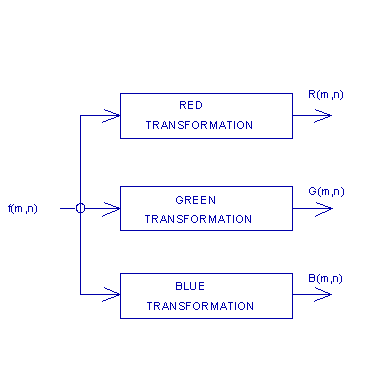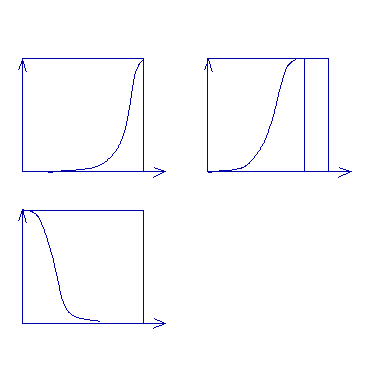6.7 Computer Experiments with XV

Color Images and Control Windows. XV automatically displays a color image as color when it is one of the color formats that it knows (GIF, TIF, JPEG, BMP, TARGA, and raw data PPM files are included). We use the sf.gif file from the same source as the other files (lena256.tif and shuttle.tif). Copy sf.gif to the personal images directory as before. Then type

/images\$ startx<ENTER>

Next, run XV with the filename sf.gif.

/images\$ xv sf.gif<ENTER>

The image displayed is a color photograph of part of old San Francisco with a view toward the Bay Bridge to Oakland/Berkeley. It is 640x422 and is in the 8-bit color mode (256 colors), of which 231 unique colors are present. Click the right mouse button with the pointer inside of the image area to obtain the xv controls window. It will display the information given above in the bottom half of this window.

Depending on the size of one's color screen, one may want to reduce the size of the displayed image by left-clicking on the Image Size bar at the top right of the xv controls window, holding the left mouse button down and dragging the pointer down to the line 10% Smaller and releasing. This can be repeated until the right size is obtained (and use 10% Larger if desired).

Move the xv controls window and the image to the top of the screen beside each other. Put the pointer on the Windows bar type button in the xv controls window, hold the left mouse button and move the pointer down to the line Color Editor and release. The large xv color editor window comes up at the bottom of the screen. It is a good idea to move it up so that it overlaps slightly the image and xv controls windows. On the upper left of the xv color editor window is a 16x16 block of color squares that is a 256-color map used in this image.

Recall that the 8-bit color mode uses 256 values (0,...,255) that are numbers (addresses) of the 256 color registers. Three n-bit values are stored in each color register (n = 6 for SVGA graphics interfaces) to represent the R, G and B values. For example, the value 000000 111111 111111 in an 18-bit color register would hold R = 0, G = 63 and B = 63, which is Cyan.

At the bottom of the xv color editor window is the intensity square. On the right are the R, G and B level squares. All of these squares contain the graphs of the form y = x with nodes for changing these identity transformations as desired (and a RESET button for resetting them back to the identity maps).

Experiments with HSI. We assume here that the user is in XV with the color image sf.gif and the xv controls window side by side at the top of the screen and the xv color editor just below them. To the top left of the xv color editor window is the color map. To the right of that is a section with three "clock" counters, each with the caption of Red, Green or Blue. Above the Blue counter is the RGB/HSV bar button. HSV is the HSI color model that we are familiar with, where "I" is called "V" for value.

We left-click the mouse on the RBG/HSV bar and the Red, Green and Blue counters immediately become the Hue, Saturation and Value counters. Click again and to return to the original setting. Observe that Red is the highest, Green is the next, and Blue is the lowest. This image is composed of warm colors.

In the middle vertical strip of the xv color editor window, center position, is a counter designated as Saturation. By clicking on the arrow buttons (or on the clock itself), we can move the "clock" hand clockwise or counter- clockwise to reduce or increase the saturation, respectively. Move it counter clockwise to the -60 position (or even the -100% position). Now examine the displayed image. It is devoid of color content because it is thoroughly diluted with white light (intensity). Now move counter clockwise to -40%. A slight tinge of color is detectable. At -36% the slight color content is more obvious and at -30% it appears in the lights on the bridge and in the lights on top of the dark building in the left foreground. At about -20% to -10% the image appears quite natural, but at 0% it is beginning to appear vivid. At 10% the image is strongly colored to the extent that the green lights appear unnatural. Continuing in this manner, the color grows more vivid at 20% and completely unreal at 30%.

RGB Experiments. Click on the RESET button at the bottom left of the window to return to the original image. Now we experiment with the intensity I = (R + G + B)/3. At the bottom center of the xv color editor window is the Intensity window. Left-click and drag the nodes on the graph to change the intensity levels. Clicking on the RESET button in the Intensity block restores the original image. We can make the contrast greater by moving the lower node downward and the upper node upward.

After resetting to get the original image back, let us change the Red transformation at the top right of the xv color editor. To strenghten the R and make the colors warmer, we use the GAM (gamma) button in the Red box (just below the reset button). We then click on GAM and type in 1.7 from the keyboard and hit <ENTER>. The graph changes to be concave and the image colors become warmer immediately. Similarly, change the GAM to 1.4 for Green and to 1.2 for Blue. This increases the intensity level overall because each of R, G and B has been increased. We account for this approximately by changing the gamma for Intensity to 0.72.

With the current color setting, we sharpen the image intensity by left-clicking on Algorithms in the upper right corner of the xv controls window, hold the mouse button down and drag the pointer down to the line Sharpen and release. Left-click on the OK button (with enhancement factor of 75). This process takes a moment, but then the sharpened image appears. To save this image, click on the Save bar of the xv controls window, then click and drag to select PS (postscript). Be sure that Color is selected and then click on OK. The sizing of the image to be saved as sf.ps is requested. We click on OK after that and the image is saved in Postscript color file format for printing to a color postscript printer.

Figure 6.10 shows the original image sf.gif, while Figure 6.11 presents the image that was processed with the gamma adjustments stated above followed by the sharpening of the intensity, which we saved as sfshrclr.ps. Figure 6.12 shows the image that results from processing the original image only by increasing to saturation using XV to +45%. From -100% to +100%, this is actually a saturation of 72.5%. These color images are shown at the end of the chapter, after the exercises that follow below.

6.8 Exercises

6.1 An golden orange color is to be used to color a graphic drawing of a moon. Using two primary colors and combining them linearly, design a color (use two of R, G and B in the proper proportions). Note that a circle can be drawn on a sheet of paper, colored yellow with a felt-tipped pen, the drawing can then be scanned into a file in a computer as a TIF, GIF, JPEG or other file and then processed with RGB gammas to obtain the desired color.

6.2 In Exercise 6.1 above, add a third primary color to add some white light to the moon color. Give a proportional combination of the colors so that the white light dilution is about 25%. Note that Saturation can be used in XV to add the white light to get the desirable effect.

6.3 Convert the following 8-bit color mode color residing in a 18-bit color register to HSI:

01010100001111011111

What color is this? What is the percentage of white light and what is the saturation?

6.4 Calculate all of the steps to arrive at Equations (6.10a,b)

6.5 Calculate all of the steps to arrive at Equations (6.11).

6.6 Derive Equation (6.13a) from Equations (6.10a,b) and (6.11).

6.7 Convert H = 30, S = 80%, I = 70% to (r,g,b) and then to (R,G,B), where each of R, G and B is between 0 and 1.

6.8 Convert the RGB colors of Figure 6.3 above to YIQ. Increase the brightness Y by 10% and then convert back to RGB. Put the RGB into the form of 18 bits for the three colors (6 bits per color) and compare to 01010100001111011111. What is the difference?

6.9 Some people leave grays near black at black and grays near white at white, but add color to shades in between, with lighter shades of gray being converted to more reddish shades of color of greater intensity and more white light dilution near the upper end (white). The darker shades are converted to more bluish colors of lesser intensity near black. What are the advantages and disadvantages of this approach?

6.10. Design a color transformation for each of R, G and B that fits with the strategy of Exercise 6.9 above. Make the transformations continuous, that is, with no jumps (see Figures 6.8 and 6.9).

6.11 Use XV to process the image sf.gif so that the colors are warmer and slightly stronger. You may use a combination of processing with parts of HSI and RGB.

6.12 Is there a way to use XV to add color to a gray image. If so, then add color to lena256.pgm.